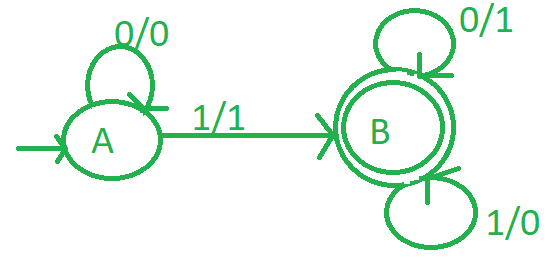Related Articles

# Design a mealy machine for 2’s complement

• Last Updated : 07 Jul, 2021

Mealy machine is a finite-state machine, its current state and the current inputs determines the output of this machine.

2’s complement :
It is the mathematical operation on binary numbers. It is used for computation as a method of signed number representation. Its complement with respect to 2N defines the two’s complement an N-bit number.

Attention reader! Don’t stop learning now. Get hold of all the important CS Theory concepts for SDE interviews with the CS Theory Course at a student-friendly price and become industry ready.

Logic:-
First calculate 1’s complement of binary number, convert 1 to 0 and 0 to 1 and then add 1 to it. For example, if binary number is 1011 then its 1’s complement is 0100 and its 2’s complement is 0101

Design mealy machine :

1. Take initial state A.
2. If there are n number of zeros at initial state, it will remain at initial state.
3. Whenever first input 1 is found then it gives output 1 and go to state B.
4. In state B, if input is zero, output will be 1.And if input is 1 then output will be 0.
5. And then set state B as final state.

The approach goes as follows:

1. Start from right to left.
2. Ignore all 0’s.
3. When 1 comes ignore it and then take 1’s complement of every digit.Figure – Mealy machine of 2’s complement

Example-1:

1. Lets take 001 and we know that its 2’s complement is (110+1 = 111).
2. So scan from right to left.
3. On state A ‘1’ came first to go to stage B and in output write 1.
4. On state B replace ‘0’ with ‘1’ and vice-versa.
5. So finally we got 111 as output.
6. Be aware that the output is also printed in right to left order.

Example-2:

1. Lets take 01 and we know that its 2’s complement is (10+1 = 11).
2. So scan from right to left.
3. On state A ‘1’ came first to go to stage B and in output write 1.
4. On state B replace ‘0’ with ‘1’ and vice-versa.
5. So finally we got 11 as output.
6. Be aware that the output is also printed in right to left order.
My Personal Notes arrow_drop_up